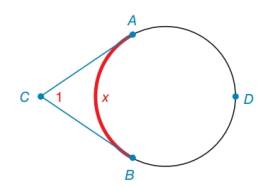Chapter 6.2, Problem 26EElementary Geometry For College St...

7th Edition
Alexander + 2 others
ISBN: 9781337614085

Solutions

Chapter
SectionElementary Geometry For College St...

7th Edition
Alexander + 2 others
ISBN: 9781337614085
Textbook Problem

Use the result from Exercise 25 to find m ∠ 1 if m A B ⌢ = 104 ° .Exercises 25, 26

To determine

To find:

The value of. m1 if mAB=104.

Explanation

Calculation:

Given, mAB=104°.

From the problem 25, if mAB=x then m1=180°x.

Since, it is given that, x=104°

Still sussing out bartleby?

Check out a sample textbook solution.

See a sample solution

The Solution to Your Study Problems

Bartleby provides explanations to thousands of textbook problems written by our experts, many with advanced degrees!

Get Started

In Exercises 2340, find the indicated limit. 28. limt3(4t22t+1)

Applied Calculus for the Managerial, Life, and Social Sciences: A Brief Approach

In Exercises 1-4, simplify the expression. x316xx3+2x28x

Calculus: An Applied Approach (MindTap Course List)

The graph of x = cos t, y = sin2 t is:

Study Guide for Stewart's Multivariable Calculus, 8th

Rewrite 10x2xdx using a trigonometric substitution. a) cos10sind b) 110sincos2d c) 10cos2sind d) 10sincosd

Study Guide for Stewart's Single Variable Calculus: Early Transcendentals, 8th